# Tim Nolet

CTO

Tim is the founder of Checkly. He used to work on big Enterprisey Java stuff almost 20 years ago but now he is vertically aligning divs most of the time. Actually studied to be an art historian.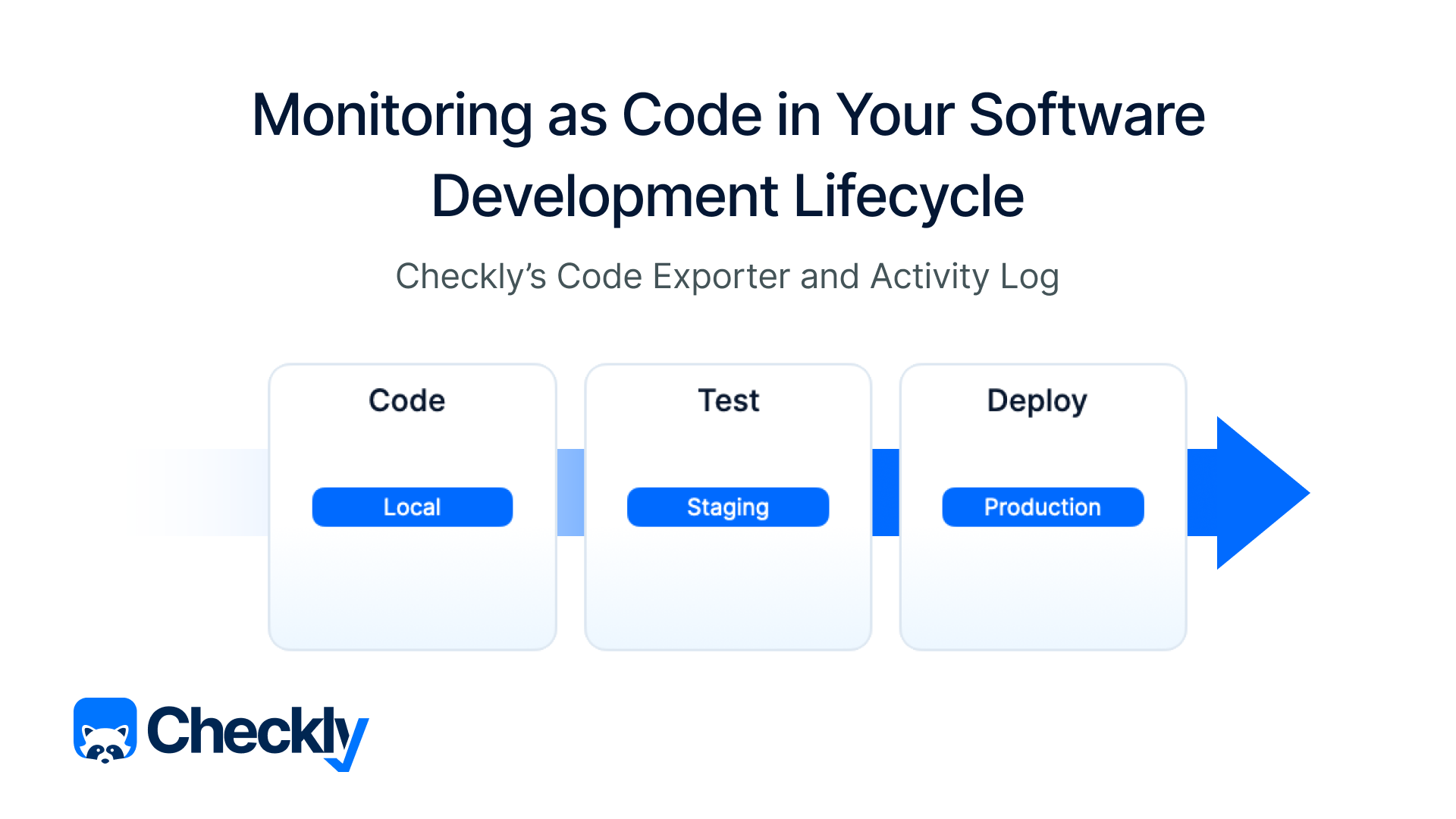## Monitoring as Code in Your Software Development Lifecycleself.__wrap_b=(t,n,e)=>{e=e||document.querySelector(`[data-br="\${t}"]`);let a=e.parentElement,r=R=>e.style.maxWidth=R+"px";e.style.maxWidth="";let o=a.clientWidth,c=a.clientHeight,i=o/2-.25,l=o+.5,u;if(o){for(;i+1<l;)u=Math.round((i+l)/2),r(u),a.clientHeight===c?l=u:i=u;r(l*n+o*(1-n))}e.__wrap_o||(typeof ResizeObserver!="undefined"?(e.__wrap_o=new ResizeObserver(()=>{self.__wrap_b(0,+e.dataset.brr,e)})).observe(a):process.env.NODE_ENV==="development"&&console.warn("The browser you are using does not support the ResizeObserver API. Please consider add polyfill for this API to avoid potential layout shifts or upgrade your browser. Read more: https://github.com/shuding/react-wrap-balancer#browser-support-information"))};self.__wrap_b(":R65l66:",1)Tim Nolet## Announcing Checkly CLI GA and Test Sessions Betaself.__wrap_b=(t,n,e)=>{e=e||document.querySelector(`[data-br="\${t}"]`);let a=e.parentElement,r=R=>e.style.maxWidth=R+"px";e.style.maxWidth="";let o=a.clientWidth,c=a.clientHeight,i=o/2-.25,l=o+.5,u;if(o){for(;i+1<l;)u=Math.round((i+l)/2),r(u),a.clientHeight===c?l=u:i=u;r(l*n+o*(1-n))}e.__wrap_o||(typeof ResizeObserver!="undefined"?(e.__wrap_o=new ResizeObserver(()=>{self.__wrap_b(0,+e.dataset.brr,e)})).observe(a):process.env.NODE_ENV==="development"&&console.warn("The browser you are using does not support the ResizeObserver API. Please consider add polyfill for this API to avoid potential layout shifts or upgrade your browser. Read more: https://github.com/shuding/react-wrap-balancer#browser-support-information"))};self.__wrap_b(":R69l66:",1)Tim Nolet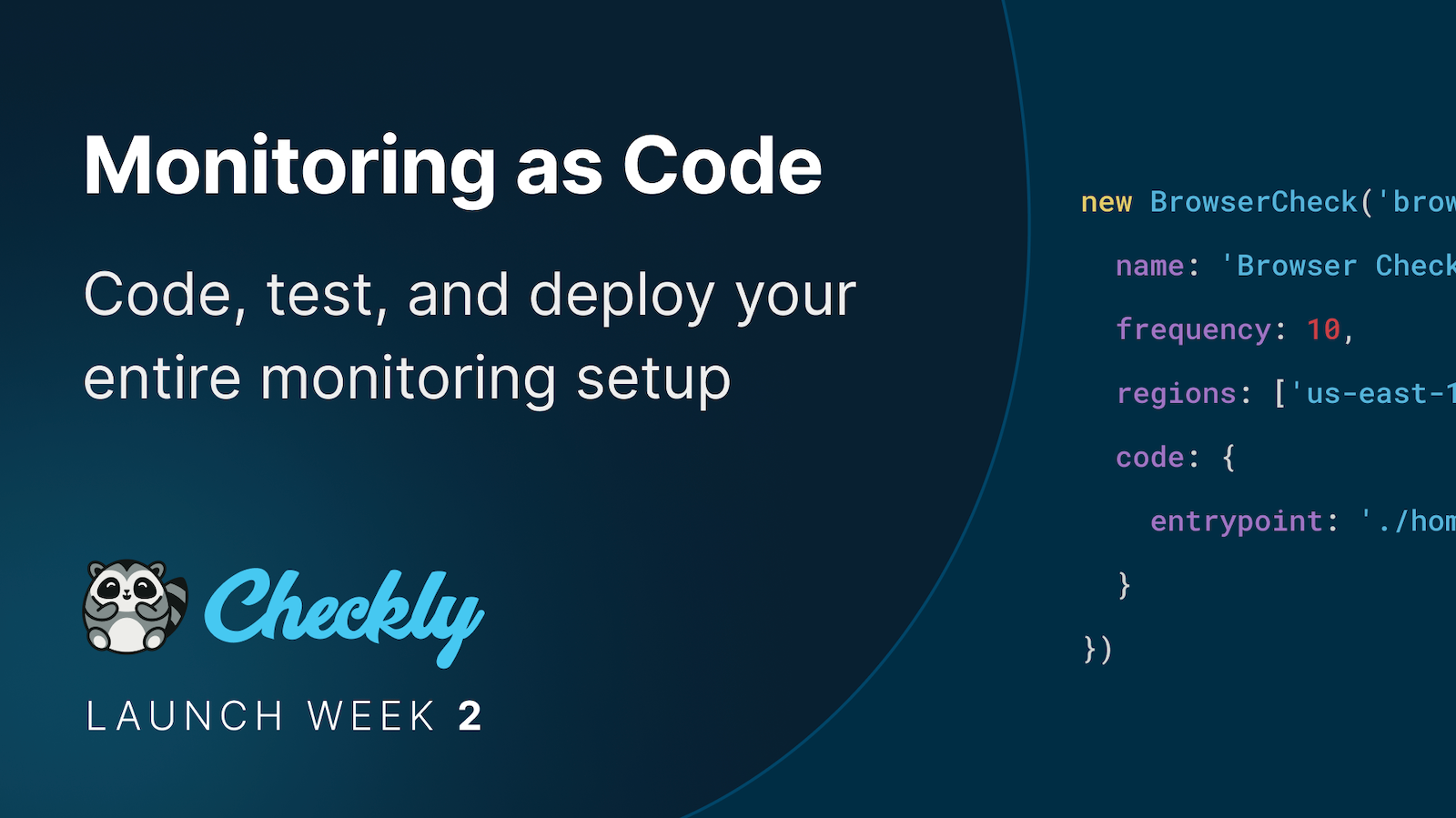## Why We Built a Developer-First Typescript CLI for Monitoring as Codeself.__wrap_b=(t,n,e)=>{e=e||document.querySelector(`[data-br="\${t}"]`);let a=e.parentElement,r=R=>e.style.maxWidth=R+"px";e.style.maxWidth="";let o=a.clientWidth,c=a.clientHeight,i=o/2-.25,l=o+.5,u;if(o){for(;i+1<l;)u=Math.round((i+l)/2),r(u),a.clientHeight===c?l=u:i=u;r(l*n+o*(1-n))}e.__wrap_o||(typeof ResizeObserver!="undefined"?(e.__wrap_o=new ResizeObserver(()=>{self.__wrap_b(0,+e.dataset.brr,e)})).observe(a):process.env.NODE_ENV==="development"&&console.warn("The browser you are using does not support the ResizeObserver API. Please consider add polyfill for this API to avoid potential layout shifts or upgrade your browser. Read more: https://github.com/shuding/react-wrap-balancer#browser-support-information"))};self.__wrap_b(":R6dl66:",1)Tim Nolet## Using Client Certificates for mTLS and Zero Trust Networksself.__wrap_b=(t,n,e)=>{e=e||document.querySelector(`[data-br="\${t}"]`);let a=e.parentElement,r=R=>e.style.maxWidth=R+"px";e.style.maxWidth="";let o=a.clientWidth,c=a.clientHeight,i=o/2-.25,l=o+.5,u;if(o){for(;i+1<l;)u=Math.round((i+l)/2),r(u),a.clientHeight===c?l=u:i=u;r(l*n+o*(1-n))}e.__wrap_o||(typeof ResizeObserver!="undefined"?(e.__wrap_o=new ResizeObserver(()=>{self.__wrap_b(0,+e.dataset.brr,e)})).observe(a):process.env.NODE_ENV==="development"&&console.warn("The browser you are using does not support the ResizeObserver API. Please consider add polyfill for this API to avoid potential layout shifts or upgrade your browser. Read more: https://github.com/shuding/react-wrap-balancer#browser-support-information"))};self.__wrap_b(":R6hl66:",1)Tim Nolet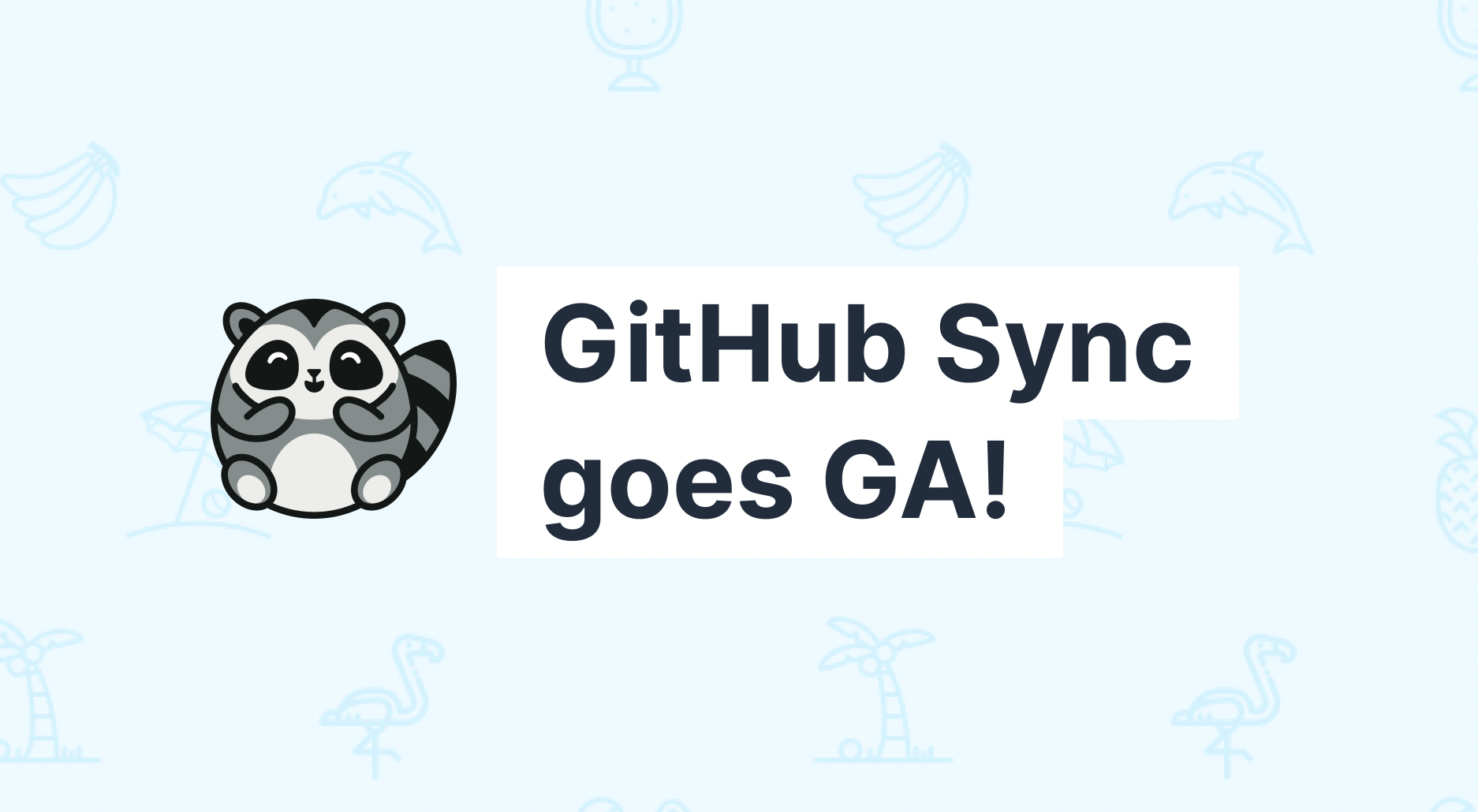## Checkly GitHub Sync Goes into GAself.__wrap_b=(t,n,e)=>{e=e||document.querySelector(`[data-br="\${t}"]`);let a=e.parentElement,r=R=>e.style.maxWidth=R+"px";e.style.maxWidth="";let o=a.clientWidth,c=a.clientHeight,i=o/2-.25,l=o+.5,u;if(o){for(;i+1<l;)u=Math.round((i+l)/2),r(u),a.clientHeight===c?l=u:i=u;r(l*n+o*(1-n))}e.__wrap_o||(typeof ResizeObserver!="undefined"?(e.__wrap_o=new ResizeObserver(()=>{self.__wrap_b(0,+e.dataset.brr,e)})).observe(a):process.env.NODE_ENV==="development"&&console.warn("The browser you are using does not support the ResizeObserver API. Please consider add polyfill for this API to avoid potential layout shifts or upgrade your browser. Read more: https://github.com/shuding/react-wrap-balancer#browser-support-information"))};self.__wrap_b(":R6ll66:",1)Tim NoletStefan Judis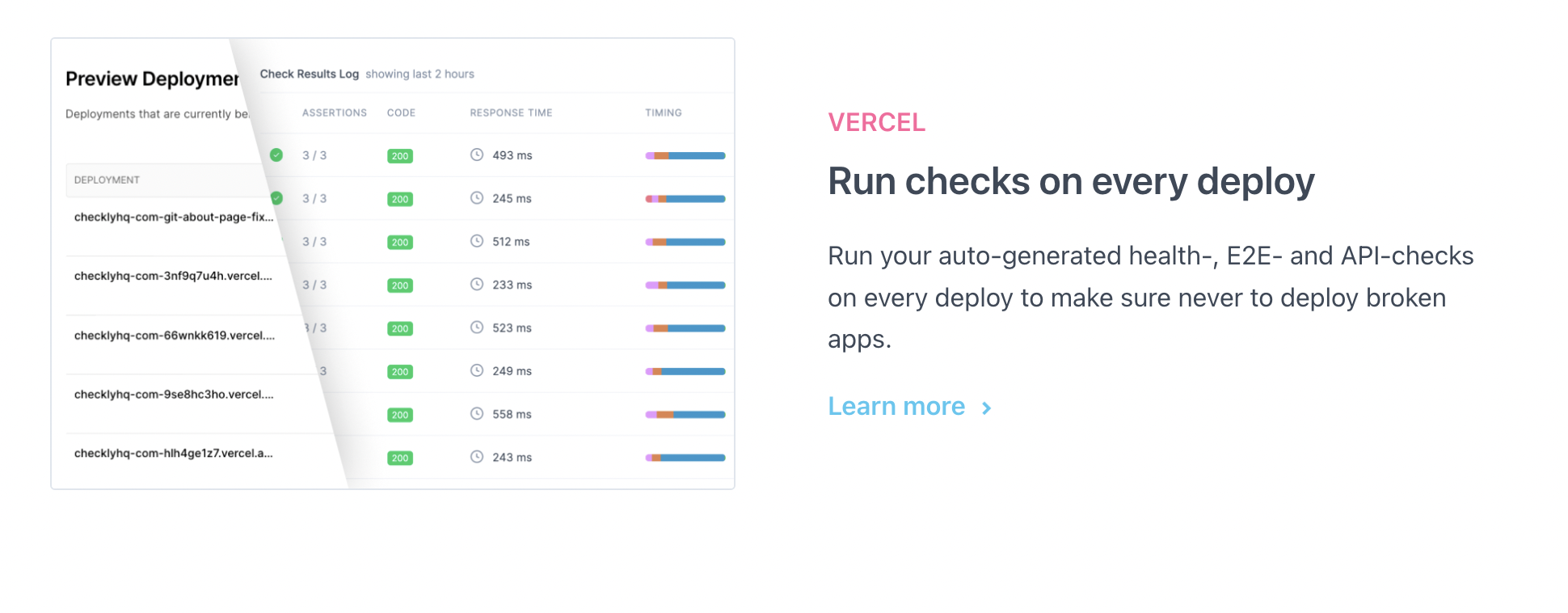## Launching Performance and Error Tracing and new Vercel integrationself.__wrap_b=(t,n,e)=>{e=e||document.querySelector(`[data-br="\${t}"]`);let a=e.parentElement,r=R=>e.style.maxWidth=R+"px";e.style.maxWidth="";let o=a.clientWidth,c=a.clientHeight,i=o/2-.25,l=o+.5,u;if(o){for(;i+1<l;)u=Math.round((i+l)/2),r(u),a.clientHeight===c?l=u:i=u;r(l*n+o*(1-n))}e.__wrap_o||(typeof ResizeObserver!="undefined"?(e.__wrap_o=new ResizeObserver(()=>{self.__wrap_b(0,+e.dataset.brr,e)})).observe(a):process.env.NODE_ENV==="development"&&console.warn("The browser you are using does not support the ResizeObserver API. Please consider add polyfill for this API to avoid potential layout shifts or upgrade your browser. Read more: https://github.com/shuding/react-wrap-balancer#browser-support-information"))};self.__wrap_b(":R6pl66:",1)Hannes LenkeTim Nolet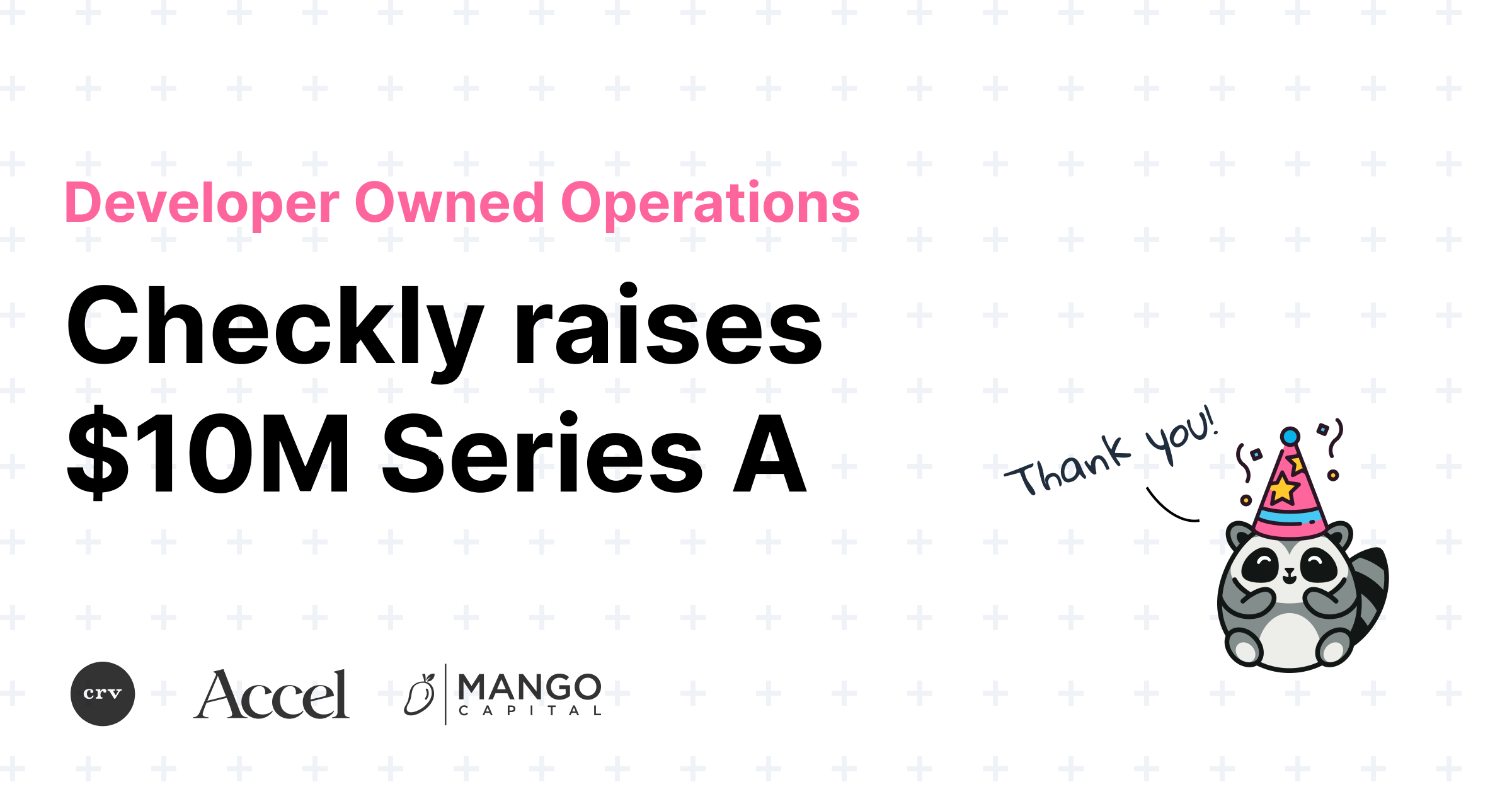## Checkly raises \$10M for Developer Owned Operationsself.__wrap_b=(t,n,e)=>{e=e||document.querySelector(`[data-br="\${t}"]`);let a=e.parentElement,r=R=>e.style.maxWidth=R+"px";e.style.maxWidth="";let o=a.clientWidth,c=a.clientHeight,i=o/2-.25,l=o+.5,u;if(o){for(;i+1<l;)u=Math.round((i+l)/2),r(u),a.clientHeight===c?l=u:i=u;r(l*n+o*(1-n))}e.__wrap_o||(typeof ResizeObserver!="undefined"?(e.__wrap_o=new ResizeObserver(()=>{self.__wrap_b(0,+e.dataset.brr,e)})).observe(a):process.env.NODE_ENV==="development"&&console.warn("The browser you are using does not support the ResizeObserver API. Please consider add polyfill for this API to avoid potential layout shifts or upgrade your browser. Read more: https://github.com/shuding/react-wrap-balancer#browser-support-information"))};self.__wrap_b(":R6tl66:",1)Hannes LenkeTim NoletTimo Euteneuer## Post mortem: browser checks failing to report resultsself.__wrap_b=(t,n,e)=>{e=e||document.querySelector(`[data-br="\${t}"]`);let a=e.parentElement,r=R=>e.style.maxWidth=R+"px";e.style.maxWidth="";let o=a.clientWidth,c=a.clientHeight,i=o/2-.25,l=o+.5,u;if(o){for(;i+1<l;)u=Math.round((i+l)/2),r(u),a.clientHeight===c?l=u:i=u;r(l*n+o*(1-n))}e.__wrap_o||(typeof ResizeObserver!="undefined"?(e.__wrap_o=new ResizeObserver(()=>{self.__wrap_b(0,+e.dataset.brr,e)})).observe(a):process.env.NODE_ENV==="development"&&console.warn("The browser you are using does not support the ResizeObserver API. Please consider add polyfill for this API to avoid potential layout shifts or upgrade your browser. Read more: https://github.com/shuding/react-wrap-balancer#browser-support-information"))};self.__wrap_b(":R71l66:",1)Tim NoletNico Domino
## Changelog: Reporting, deployments and many, many enhancements and fixesself.__wrap_b=(t,n,e)=>{e=e||document.querySelector(`[data-br="\${t}"]`);let a=e.parentElement,r=R=>e.style.maxWidth=R+"px";e.style.maxWidth="";let o=a.clientWidth,c=a.clientHeight,i=o/2-.25,l=o+.5,u;if(o){for(;i+1<l;)u=Math.round((i+l)/2),r(u),a.clientHeight===c?l=u:i=u;r(l*n+o*(1-n))}e.__wrap_o||(typeof ResizeObserver!="undefined"?(e.__wrap_o=new ResizeObserver(()=>{self.__wrap_b(0,+e.dataset.brr,e)})).observe(a):process.env.NODE_ENV==="development"&&console.warn("The browser you are using does not support the ResizeObserver API. Please consider add polyfill for this API to avoid potential layout shifts or upgrade your browser. Read more: https://github.com/shuding/react-wrap-balancer#browser-support-information"))};self.__wrap_b(":R75l66:",1)Tim Nolet